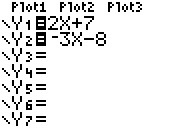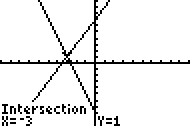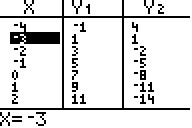# Knowledge Base

## Solution 17089: Solving a System of Linear Equations Using a Graph on the TI-83 Family, TI-84 Plus Family and TI-Nspire™ Handheld in TI-84 Plus Mode.

### How do I use a graph to solve a system of linear equations on the TI-83 family, TI-84 Plus family and TI-Nspire handheld in TI-84 Plus mode?

To solve a system of linear equations using a graph on the TI-83 family, TI-84 Plus family and TI-Nspire handheld in TI-84 Plus mode, follow the example below. The purpose is to solve a system of two equations and two unknowns.

Example: Using a graph, find the solution for the equations y = 2x + 7 and y = -3x - 8.

• Press [Y=] to access the Y= editor.
• For Y1, input 2X+7.
• For Y2 input -3X-8.• Press [GRAPH] to graph the equations.
• Press [2nd] [TRACE]  to select the "intersect" command from the CALC menu.
• The handheld will prompt for the "First curve" which means it wants the user to select the first equation. Press [ENTER] to mark the first equation.

NOTE: Either the Y1 equation or the Y2 equation can be marked as the first equation.

• The handheld will prompt for the "Second curve" which means it wants the user to select the second equation. The handheld should also automatically jump to the second equation. Press [ENTER] to mark the second equation.
• The handheld will prompt for the "Guess?" which means it wants the user to take a guess at the solution. Using the [] and [] keys, move the cursor as close as possible to the intersection point (which is the solution) and press [ENTER]. The handheld will display the solutions for both the X and Y variables as a coordinate point (-3,1).• To check the accuracy of the solution, access the TABLE by pressing [2nd] [GRAPH]. From the X column, use the [] and [] keys to scroll and locate the value (-3). When X=-3, both Y1 and Y2 are equal to 1 which means the solution is accurate.Please see the TI-83 family and TI-84 Plus family guidebooks for additional information.

TI-Nspire handheld in TI-84 Plus mode users may refer to the TI-84 Plus family guidebook.